# Let's Get Started

You are going to experiment by building a Spaghetti Bridge to gather data. Alternatively, you can use the data provided. You will use the data to create a graph and a line of best fit in order to make predictions. Before you get started, don't forget to print out the OnTRACK Algebra Journal for this resource.

TEKS Standards and Student Expectations

A(4) Linear functions, equations, and inequalities. The student applies the mathematical process standards to formulate statistical relationships and evaluate their reasonableness based on real-world data. The student is expected to:

A(4)(A) calculate, using technology, the correlation coefficient between two quantitative variables and interpret this quantity as a measure of the strength of the linear association

A(4)(B) compare and contrast association and causation in real-world problems

A(4)(C) write, with and without technology, linear functions that provide a reasonable fit to data to estimate solutions and make predictions for real-world problems

Resource Objective(s)

Given an experimental situation, write linear functions that provide a reasonable fit to data to estimate the solutions and make predictions.

Essential Questions

What is the purpose of creating a line of best fit?

How can graphs be used to make predictions?

Vocabulary

# Introduction to Experimentation

When you experiment and collect data, the data sets can be analyzed. The data for those variables can be plotted on a graph, and you can come up with a line of best fit. You can then make predictions based on the line of best fit.

An example of an experiment in which data can be collected is a Spaghetti Bridge. Using a spaghetti bridge, we can compare the number of strands of spaghetti to the number of marbles that it would hold.

These pictures show a Spaghetti Bridge that someone has made and suspended between two chairs. If you would like to conduct the Spaghetti Bridge experiment, the directions can be found below the pictures. If you do not have the materials to make a Spaghetti Bridge, you can still work with data that someone else has collected. For the Journal Activity, we will provide data collected by someone else for a Spaghetti Bridge.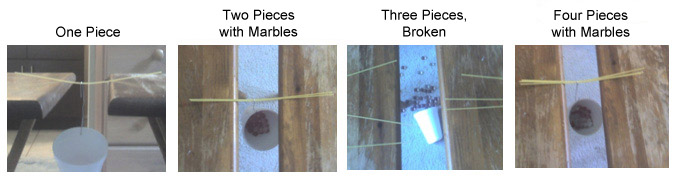Spaghetti Bridge Experiment

You will need a paper clip, paper/styrofoam cup, 20 pieces of uncooked spaghetti, two chairs, and 100 equally weighted marbles.

Procedure

1. Arrange the chairs so a spaghetti bridge can be formed.
2. A typical piece of spaghetti is about 24 cm long. Leave approximately 8 cm of spaghetti on each chair. You may modify, but keep the arrangement consistent.
3. Use the paperclip to hook the cup onto the spaghetti bridge.
4. Place a marble in the cup, one at a time, until the spaghetti breaks.
5. Wait 5 seconds between adding each marble. The spaghetti may not break instantly, but it will eventually succumb to the pressure.
6. Copy the data into the table into your notes.
Number of Pieces of Spaghetti Number of Marbles to Break Spaghetti
1
2
3
4

Later you will use data to predict the answers to questions, such as how many marbles a 10 spaghetti bridge could hold.

# Spaghetti Bridge Data

If you did not get to make the Spaghetti Bridge, you can still work with data that someone else has collected. This table shows the data for a Spaghetti Bridge.

Number of Pieces of Spaghetti Number of Marbles to Break Spaghetti
1 18
2 40
3 56
4 70

This data will be used to make predictions, such as how many marbles a 10 spaghetti bridge could hold.

Go to the linked interactive webpage for the Regression Activity and input the Spaghetti Bridge data into the empty box below the interactive graph, using the following instructions.

1. Enter the numbers from the table into the empty box below the graph. Remember to put a comma between the number of pieces of spaghetti and the number of marbles.
2. After each pair of numbers, click "Enter."
3. After entering all four pairs of numbers, click "Update Plot."
4. When your points are plotted, you can check the box labeled "Display line of best fit" to see the scatterplot with the line graphed and the equation for the line of best fit.

The line of best fit is the closest linear approximation of the data. This equation can now be used to estimate how many marbles 10 pieces of spaghetti would hold. Your activity page should now look something like the following image.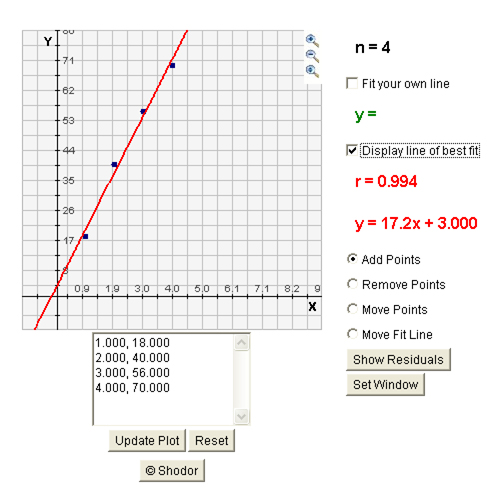To estimate the number of marbles, you can substitute 10 in for the pieces of spaghetti (x-value) or you can reset the graph by pressing "Set Window."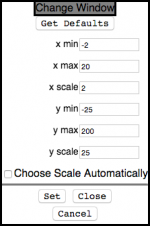The "Change Window" will open (as shown on the right) to set the x- and y-values for the graph. Since you know you want to see at least 10, set the x-value for 12 and set the y-value for a higher number.  Click "Set" at the bottom to change the window, and then click "Close" to see your graph. Can you see the value for 10? If not, set the window again to a larger y-value. You must uncheck "Choose Scale Automatically" if you set your own scale.

You may want to use the following values:

x min = -2
x max = 20
x scale = 2
y min = -25
y max = 200
y scale = 25

# Finding a Line of Best Fit

Let us focus for a moment on finding the line of best fit for a data set. You can use the same interactive Regression Activity webpage as before to find the line of best fit. Is there a relationship between the fat grams and the total calories in fast food?

 Sandwich Total Fat (g) Total Calories Hamburger 18 260 Cheeseburger 40 320 Quarter Pound Burger 56 420 Quarter Pound Burger with Cheese 70 530 Specialty Burger 31 560 Sandwich Special 31 550 Sandwich Special with Bacon 34 590 Crispy Chicken Nuggets 25 500 Fish Sandwich 28 560 Grilled Chicken 20 440 Grilled Chicken Light 5 300

Click on the link below for the Regression Activity.

1. Put the points from the sandwich table into the graph.
2. Select "Move Fit Line."
3. Check the "Fit Your Own Line" box.
4. Move the green points until you think you have the line of best fit. (The green line shown below is not close enough.)
5. Once you think you have the line of best fit, check the "Display Line of Best Fit" box.

Using your notes, green equations, and red equations, predict the number of calories that would be in a sandwich containing 40 grams of fat.

# Using a Graphing Calculator

You can do this same activity using your graphing calculator. We'll use these data first since you've used it before.

Sandwich Total Fat (g) Total Calories
Hamburger 18 260
Cheeseburger 40 320
Quarter Pounder 56 420
Quarter Pounder with Cheese 70 530
Big Mac 31 560
Arch Sandwich Special 31 550
Arch Special with Bacon 34 590
Crispy Chicken 25 500
Fish Fillet 28 560
Grilled Chicken 20 440
Grilled Chicken Light 5 300

Think about the steps you would take to plot these points using paper and pencil. You have to take similar steps on the calculator.

1. First press the Stat button. Be sure that Edit is chosen and press Enter.
2. Input the data in list 1 and list 2. Put the Total Fat in L1 and Total Calories in L2.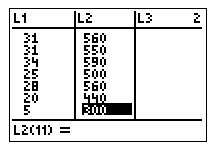3. Press 2nd and Y= to turn on Plot 1. Press Graph. Be sure that the scatterplot graph is selected and that it is set to Xlist:L1 and Ylist:L2. Then press Graph.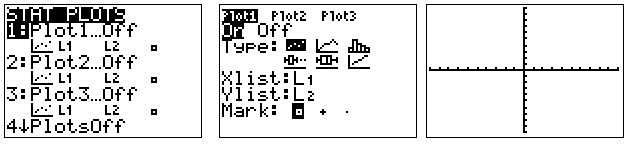4. If you do not see a graph, it's probably because the window is not set correctly for the data. Look at the data. L1 has data from 5 to 34 and L2 has data from 260 to 590. Adjust the Window and Graph.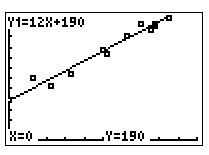5. Input the data in list 1 and list 2. Put the Total Fat in L1 and Total Calories in L2.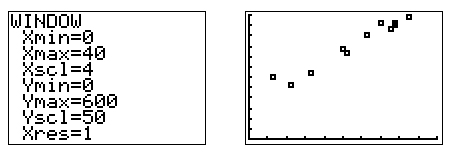Now it will appear like the applet. Here is one possible result.